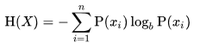Skip to content
Related Articles
Information Entropy using MATLAB
• Difficulty Level : Expert
• Last Updated : 18 Aug, 2020

Information entropy is the aggregate rate at which information is created by a stochastic wellspring of information. The proportion of information entropy related to every potential information value is the negative logarithm of the likelihood mass function for the worth. Hence, when the information source has lower likelihood esteem(i.e., when a low-likelihood occasion happens), the occasion conveys more data than when the source information has higher-likelihood esteem. The measure of data passed on by each occasion characterized along these lines turns into a random variable whose expected worth is the information entropy. Entropy is zero when one result is sure to happen.

Formula:Example 1 : A discrete memoryless source i.e. DMS ‘X’ has 4 symbols x1, x2, x3 and x4 with probabilities P(x1) = 0.333, P(x2) = 0.333, P(x3) = 0.167 and P(x4) = 0.167.
So, H(X) = -0.333 log2(0.333)-0.333 log2(0.333)-0.167 log2(0.167)-0.167 log2(0.167)
H(X) = 1.918

Example 2 : A discrete memoryless source i.e. DMS ‘X’ has 2 symbols x1 and x2 with probabilities P(x1) = 0.600 and P(x2) = 0.400
So, H(X) = -0.600 log2(0.600)-0.400 log2(0.400)
H(X) = 0.970

Here is the MATLAB code to calculate the information entropy of a string.

 `clc;`` ` `# the string``x = ``'GeeksforGeeks'`` ` `# length of the string``len = length(x);``display(len);`` ` `# unique characters``u = unique(x);``display(u);`` ` `# length of unique character string``lenChar = length(u);``display(lenChar);`` ` `# creating 2 zero vectors``z = zeros(1, lenChar);``p = zeros(1, lenChar);`` ` `# finding the values of probability``for` `i = 1 : lenChar``   ``z(i) = length(findstr(x, u(i)));``   ``p(i) = z(i) / len;``end``display(z);``display(p);`` ` `# information entropy``H = 0;``for` `i=1:lenChar``  ``H = H + (-p(i) * log2(p(i)));``end``display(H);`
```x = GeeksforGeeks
len =  13
u = Gefkors
lenChar =  7
z =

2   4   1   2   1   1   2

p =

0.153846   0.307692   0.076923   0.153846   0.076923   0.076923   0.153846

H =  2.6235
```
My Personal Notes arrow_drop_up